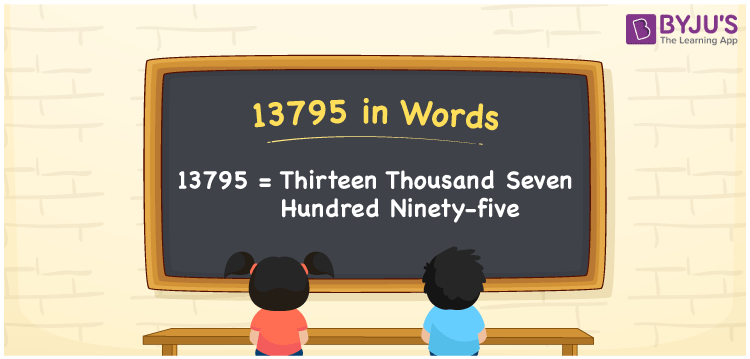# 13795 in Words

We can write 13795 in words as Thirteen thousand seven hundred ninety-five. If you bought a new steel swing for Rs. 13795, then you can say, “I bought a new steel swing for Thirteen thousand seven hundred ninety-five rupees”. Let’s understand how to convert the cardinal number 13795 into words here in this article.

 13795 in words Thirteen thousand seven hundred ninety-five Thirteen thousand seven hundred ninety-five in Numbers 13795

## 13795 in English Words

Generally, we use the English alphabet to express numbers in words. Thus, we can spell 13795 in English words as “Thirteen thousand seven hundred ninety-five”.## How to Write 13795 in Words?

In this section, you will learn how to convert the number 13795 to words using a place value chart with five columns as explained below.

 Ten thousands Thousands Hundreds Tens Ones 1 3 7 9 5

Here, ones = 5, tens = 9, hundreds = 7, thousands = 3, ten thousands = 1

Let us expand these digits as shown below.

1 × Ten thousand + 3 × Thousand + 7 × Hundred + 9 × Ten + 5 × One

= 1 × 10000 + 3 × 1000 + 7 × 100 + 9 × 10 + 5 × 1

= 10000 + 3000 + 700 + 90 + 5

= Ten Thousand + Three thousand + Seven hundred + Ninety + Five

= Thirteen thousand + Seven hundred ninety-five {since ten + three = thirteen}

= Thirteen thousand seven hundred ninety-five

Therefore, 13795 in words = Thirteen thousand seven hundred ninety-five

13795 is a natural number that is the successor of 13794 and predecessor of 13796.

13795 in words – Thirteen thousand seven hundred ninety-five

Is 13795 an even number? – No

Is 13795 an odd number? – Yes

Is 13795 a prime number? – No

Is 13795 a composite number? – Yes

Is 13795 a perfect square number? – No

Is 13795 a perfect cube number? – No

## Frequently Asked Questions on 13795 in Words

### How do you say 13795 in words?

You can spell the number 13795 in English words as Thirteen thousand seven hundred ninety-five.

### How to write Rs. 13795 in words on a cheque?

On a cheque, we can write Rs. 13795 in words as “Thirteen thousand seven hundred ninety-five rupees only”.

### What is the number name of 13795?

The number name of 13795 is Thirteen thousand seven hundred ninety-five.